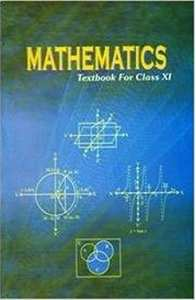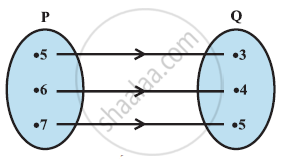Advertisement Remove all ads

# NCERT solutions for Class 11 Mathematics Textbook chapter 2 - Relations and Functions [Latest edition]

#### ChaptersAdvertisement Remove all ads
Advertisement Remove all ads
Advertisement Remove all ads

## Chapter 2: Relations and Functions

Exercise 2.1OthersExercise 2.2Exercise 2.3Exercise 2.4
Advertisement Remove all ads
Exercise 2.1[Pages 33 - 34]

### NCERT solutions for Class 11 Mathematics Textbook Chapter 2 Relations and FunctionsExercise 2.1[Pages 33 - 34]

Exercise 2.1 | Q 1 | Page 33

If (x/3+1, y-2/3)=(5/3,1/3),find the values of x and y.

Exercise 2.1 | Q 2 | Page 33

If the set A has 3 elements and the set B = {3, 4, 5}, then find the number of elements in (A × B)?

Q 2.1 | Page 33

if (x/3 + 1, y - 2/3) = (5/3, 1/3) find the values of x and y.

Exercise 2.1 | Q 3 | Page 33

If G = {7, 8} and H = {5, 4, 2}, find G × H and H × G.

Q 4.1 | Page 33

State whether the following statement are true or false. If the statement is false, rewrite the given statement correctly.

If P = {mn} and Q = {nm}, then P × Q = {(mn), (nm)}.

Exercise 2.1 | Q 4.1 | Page 33

State whether of  the statement is true or false. If the statement is false, re-write the given statement correctly:

If P = {m, n} and Q = {n, m}, then P × Q = {(m, n), (n, m)}

• True

• False

Q 4.2 | Page 33

State whether the following statement are true or false. If the statement is false, rewrite the given statement correctly.

If A and B are non-empty sets, then A × B is a non-empty set of ordered pairs (xy) such that x ∈ A and y ∈ B.

Exercise 2.1 | Q 4.2 | Page 33

State whether of  the statement is true or false. If the statement is false, re-write the given statement correctly:

If A and B are non-empty sets, then A × B is a non-empty set of ordered pairs (x, y) such that x ∈ B and y ∈ A.

• True

• False

Q 4.3 | Page 33

State whether the following statement are true or false. If the statement is false, rewrite the given statement correctly.

If A = {1, 2}, B = {3, 4}, then A × (B ∩ Φ) = Φ.

Exercise 2.1 | Q 4.3 | Page 33

State whether of  the statement is true or false. If the statement is false, re-write the given statement correctly:

(iii) If A = {1, 2}, B = {3, 4}, then A × (B ∩ ϕ) = ϕ.

• True

• False

Exercise 2.1 | Q 5 | Page 33

If A = {–1, 1}, find A × A × A.

Exercise 2.1 | Q 6 | Page 33

If A × B = {(a, x), (a, y), (b, x), (b, y)}. Find A and B.

Exercise 2.1 | Q 7.1 | Page 33

Let A = {1, 2}, B = {1, 2, 3, 4}, C = {5, 6} and D = {5, 6, 7, 8}. Verify that A × (B ∩ C) = (A × B) ∩ (A × C)

Exercise 2.1 | Q 7.2 | Page 33

Let A = {1, 2}, B = {1, 2, 3, 4}, C = {5, 6} and D = {5, 6, 7, 8}. Verify that   A × C is a subset of B × D

Exercise 2.1 | Q 8 | Page 33

Let A = {1, 2} and B = {3, 4}. Write A × B. How many subsets will A × B have? List them.

Exercise 2.1 | Q 9 | Page 33

Let A and B be two sets such that n(A) = 3 and n (B) = 2. If (x, 1), (y, 2), (z, 1) are in A × B, find A and B, where x, y and z are distinct elements.

Exercise 2.1 | Q 10 | Page 34

The Cartesian product A × A has 9 elements among which are found (–1, 0) and (0, 1). Find the set A and the remaining elements of A × A.

Exercise 2.2[Pages 35 - 36]

### NCERT solutions for Class 11 Mathematics Textbook Chapter 2 Relations and FunctionsExercise 2.2[Pages 35 - 36]

Exercise 2.2 | Q 1 | Page 35

Let A = {1, 2, 3, … , 14}. Define a relation R from A to A by R = {(x, y): 3x – y = 0, where x, y ∈ A}. Write down its domain, codomain and range.

Exercise 2.2 | Q 2 | Page 36

Define a relation R on the set N of natural numbers by R = {(xy): y = x + 5, x is a natural number less than 4; xy ∈ N}. Depict this relationship using roster form. Write down the domain and the range.

Exercise 2.2 | Q 3 | Page 36

A = {1, 2, 3, 5} and B = {4, 6, 9}. Define a relation R from A to B by R = {(xy): the difference between x and y is odd; x ∈ A, ∈ B}. Write R in roster form.

Exercise 2.2 | Q 4 | Page 36

The given figure shows a relationship between the sets P and Q. write this relation

(i) in set-builder form (ii) in roster form.

What is its domain and range?Exercise 2.2 | Q 5 | Page 36

Let A = {1, 2, 3, 4, 6}. Let R be the relation on A defined by {(ab): ab ∈ A, b is exactly divisible by a}.

(i) Write R in roster form

(ii) Find the domain of R

(iii) Find the range of R.

Exercise 2.2 | Q 6 | Page 36

Determine the domain and range of the relation R defined by R = {(xx + 5): x ∈ {0, 1, 2, 3, 4, 5}}.

Exercise 2.2 | Q 7 | Page 36

Write the relation R = {(xx3): is a prime number less than 10} in roster form.

Exercise 2.2 | Q 8 | Page 36

Let A = {xy, z} and B = {1, 2}. Find the number of relations from A to B.

Exercise 2.2 | Q 9 | Page 36

Let R be the relation on Z defined by R = {(ab): ab ∈ Z– b is an integer}. Find the domain and range of R.

Exercise 2.3[Page 44]

### NCERT solutions for Class 11 Mathematics Textbook Chapter 2 Relations and FunctionsExercise 2.3[Page 44]

Exercise 2.3 | Q 1 | Page 44

Which of the following relations are functions? Give reasons. If it is a function, determine its domain and range.

(i) {(2, 1), (5, 1), (8, 1), (11, 1), (14, 1), (17, 1)}

(ii) {(2, 1), (4, 2), (6, 3), (8, 4), (10, 5), (12, 6), (14, 7)}

(iii) {(1, 3), (1, 5), (2, 5)}

Exercise 2.3 | Q 2.1 | Page 44

Find the domain and range of the given real function:

f(x) = –|x|

Exercise 2.3 | Q 2.2 | Page 44

Find the domain and range of the following real function:

f(x) = sqrt(9 - x^2)

Exercise 2.3 | Q 3 | Page 44

A function f is defined by f(x) = 2x – 5. Write down the values of

(i) f(0), (ii) f(7), (iii) f(–3)

Exercise 2.3 | Q 4 | Page 44

The function ‘t’ which maps temperature in degree Celsius into temperature in degree Fahrenheit is defined by t(C) = "9C"/5 + 32

Find

(i) t(0)

(ii) t(28)

(iii) t(–10)

(iv) The value of C, when t(C) = 212.

Exercise 2.3 | Q 5.1 | Page 44

Find the range of each of the following functions.

f(x) = 2 – 3xx ∈ Rx > 0.

Exercise 2.3 | Q 5.2 | Page 44

Find the range of each of the following functions.

f(x) = x2 + 2, x, is a real number.

Exercise 2.3 | Q 5.2 | Page 44

Find the range of each of the following functions.

f(x) = xx is a real number

Exercise 2.4[Pages 46 - 47]

### NCERT solutions for Class 11 Mathematics Textbook Chapter 2 Relations and FunctionsExercise 2.4[Pages 46 - 47]

Exercise 2.4 | Q 1 | Page 46

The relation f is defined by f(x) = {(x^2,0<=x<=3),(3x,3<=x<=10):}

The relation g is defined by  g(x) = {(x^2, 0 <= x <= 2),(3x,2<= x <= 10):}

Show that f is a function and g is not a function.

Exercise 2.4 | Q 2 | Page 46

If f(x) = x2, find (f(1.1) - f(1))/((1.1 - 1))

Exercise 2.4 | Q 3 | Page 46

Find the domain of the function  f(x) = (x^2 + 2x + 1)/(x^2 - 8x + 12)

Exercise 2.4 | Q 4 | Page 46

Find the domain and the range of the real function f defined by f(x)=sqrt((x-1))

Exercise 2.4 | Q 5 | Page 46

Find the domain and the range of the real function f defined by f (x) = |x – 1|.

Exercise 2.4 | Q 6 | Page 46

Let f = {(x, x^2/(1+x^2)):x ∈ R} be a function from R into R. Determine the range of f.

Exercise 2.4 | Q 7 | Page 46

Let fgR → R be defined, respectively by f(x) = + 1, g(x) = 2x – 3. Find f + gf – g and f/g

Exercise 2.4 | Q 8 | Page 46

Let = {(1, 1), (2, 3), (0, –1), (–1, –3)} be a function from Z to Z defined by f(x) = ax + b, for some integers ab. Determine ab.

Exercise 2.4 | Q 9 | Page 46

Let R be a relation from N to N defined by R = {(ab): ab ∈ N and a = b2}. Are the following true?

(i) (aa) ∈ R, for all a ∈ N

(ii) (ab) ∈ R, implies (ba) ∈ R

(iii) (ab) ∈ R, (bc) ∈ R implies (ac) ∈ R.

Justify your answer in each case.

Exercise 2.4 | Q 10 | Page 46

Let A = {1, 2, 3, 4}, B = {1, 5, 9, 11, 15, 16} and = {(1, 5), (2, 9), (3, 1), (4, 5), (2, 11)}. Are the following true?

(i) f is a relation from A to B (ii) f is a function from A to B.

Justify your answer in each case.

Exercise 2.4 | Q 11 | Page 47

Let f be the subset of Z × Z defined by = {(aba + b): ab ∈ Z}. Is f a function from Z to Z: justify your answer.

Exercise 2.4 | Q 12 | Page 47

Let A = {9, 10, 11, 12, 13} and let f: A → N be defined by f(n) = the highest prime factor of n. Find the range of f.

## Chapter 2: Relations and Functions

Exercise 2.1OthersExercise 2.2Exercise 2.3Exercise 2.4## NCERT solutions for Class 11 Mathematics Textbook chapter 2 - Relations and Functions

NCERT solutions for Class 11 Mathematics Textbook chapter 2 (Relations and Functions) include all questions with solution and detail explanation. This will clear students doubts about any question and improve application skills while preparing for board exams. The detailed, step-by-step solutions will help you understand the concepts better and clear your confusions, if any. Shaalaa.com has the CBSE Class 11 Mathematics Textbook solutions in a manner that help students grasp basic concepts better and faster.

Further, we at Shaalaa.com provide such solutions so that students can prepare for written exams. NCERT textbook solutions can be a core help for self-study and acts as a perfect self-help guidance for students.

Concepts covered in Class 11 Mathematics Textbook chapter 2 Relations and Functions are Cartesian Product of Sets, Brief Review of Cartesian System of Rectanglar Co-ordinates, Relation, Concept of Functions, Some Functions and Their Graphs, Algebra of Real Functions, Ordered Pairs, Equality of Ordered Pairs, Pictorial Diagrams, Graph of Function, Pictorial Representation of a Function, Exponential Function, Logarithmic Functions.

Using NCERT Class 11 solutions Relations and Functions exercise by students are an easy way to prepare for the exams, as they involve solutions arranged chapter-wise also page wise. The questions involved in NCERT Solutions are important questions that can be asked in the final exam. Maximum students of CBSE Class 11 prefer NCERT Textbook Solutions to score more in exam.

Get the free view of chapter 2 Relations and Functions Class 11 extra questions for Class 11 Mathematics Textbook and can use Shaalaa.com to keep it handy for your exam preparation

Advertisement Remove all ads
Share
Notifications

View all notifications

Forgot password?